﻿ Nexel

# NEXEL (Formula Editor)

## Overview

Users familiar with spreadsheet-like tool can benefit of a wide library of formulas (Nexel), glued on the top of the reporting layer. This intuitive tool provides calculation capabilities on column, referring to single cells or range of cells. Once calculation rules have been defined they are applicable runtime or in a procedure command. This function can then combine the flexibility and the versatility of a spreadsheet with the power and robustness of a multidimensional object as the data-view.

## Enabling NexelFormulas

Calculation are based on Nexel Formulas. Formulas are equations that can perform calculations, return information, manipulate the contents of other cells, test conditions, and more. A formula always starts with an equal sign (=). Formulas can be enabled on blocks with:

-          Info-cube

-          Algorithm

-          Entities & Relationship

The result of the Nexel Formula overlaps the Value of the Layout Layer if any.

To enable Nexel Formulas in a block, select in the Tab Functions > “Formulas In" property, the Rule Type as following: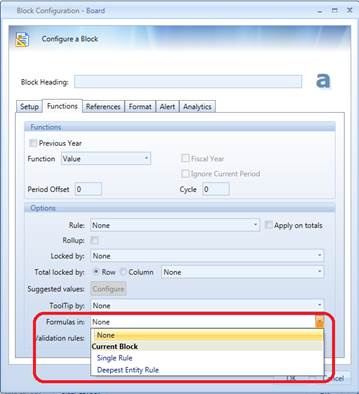NOTES

Nexel allows columns until ZZ

## Rule Type

The Rule Type defines how the Formula will be propagated through the cells of the related block.

Nexel allows to define two different Rule Types:

·         Single Rule: the Formula is the same for all the cells of the block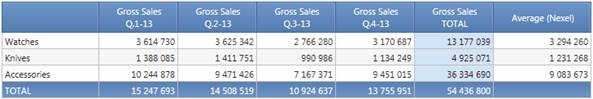In the example above, Nexel calculates for each row the Average Value of the block a (Gross Sales), and the formula is the same for all the rows.

·         Deepest Entity Rule: Each cell of the block can have a different Formula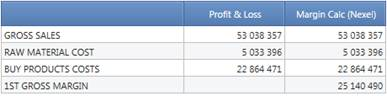In this example: 1st Gross Margin is calculated as difference between Gross Sales, Raw Material Costs and Buy Product Costs.

## Writing NexelFormulas

Nexel Formulas can be created and edit in the Formula Editor Panel.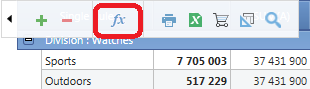1.       Double click in the cell. The cell background becomes green: this means is now possible to insert the formula.

2.       Click the cell, or press the arrow keys to move to the cell you want to use in the Formula

3.       The formula editor automatically creates the Formula with the Cell or Range coordinates and preview it

4.       By clicking on the green arrow, the formula is copied in the Formula Field.

5.       Once copied, is possible to edit the formula manually.

6.       Click on Save. The result is applied to the Report.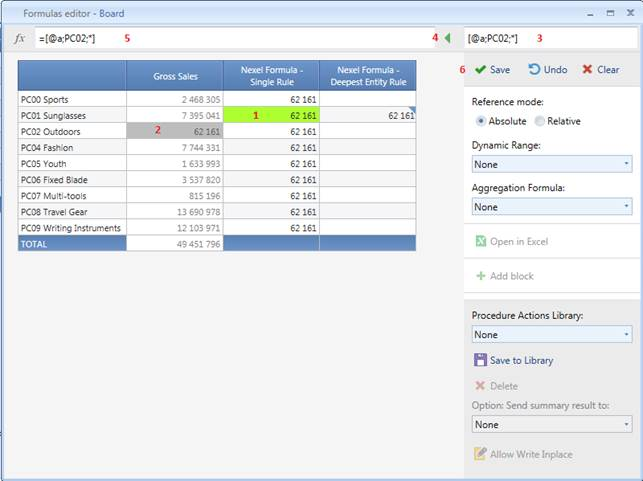NOTES

In the Formula Editor is possible to see a preview of the final result of the Nexel Layer. After the Save Button is clicked, the model is applied to the Layout.

NOTES

In the Above example we can better understand the difference between the Rule Type: Single Rule and Deepest Entity Rule.

In the 2nd and 3rd block the same formula has been written, but the result is different; in the first case the result of the formula is written in all the rows of the block; in the second case the result is written only in the cell that contains the formula.

Syntax (Cell)

Nexel Formulas must be written following this syntax :

= F(X) ([@Block; Row; Column])

F(X): Function. Defines what kind of calculation is applied (e.g. SUM, AVERAGE, MIN, MAX …). If omitted it takes by default the SUM operator.

Block: Block Identifier in the Layout (e.g. @a means Block “a?)

Row: Item’s Code of the by row Entity (e.g. P01). The symbol * means same position (row) of the cell where the formula is edit.

Column: Item’s Code of the by column Entity (e.g. 201401). The symbol * means same position (column) of the cell where the formula is edit.

NOTES

A cell is uniquely identified by the code of the the item by row and/or column even if the report displays the Description of such Item.

Syntax (Range)

Nexel Formulas must be written following this syntax :

= F(X) ([@Block; Range(cell_1:cell_2)])

F(X): Function. Defines what kind of calculation is applied (e.g. SUM, AVERAGE, MIN, MAX …). If omitted it takes by default the SUM operator.

Range: Range of cells form the left top cell to the right bottom cell.

NOTES

Ranges can be created on a single block only. They can’t collect cells from multiple blocks.

Some samples: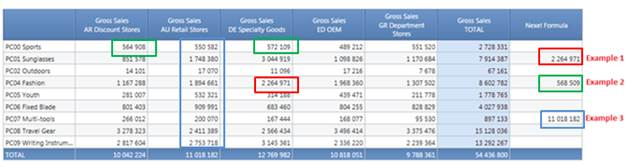Example 1

=[@a;PC04;DE]   Block a (Gross Sales); Row PC04; Column DE  2 264 971

Example 2

=([@a;PC00;AR]+[@a;PC00;DE])/2   It sums the value in Block a (Gross Sales), Row PC00, Column AR to the value in Block a (Gross Sales); Row PC00; Column DE then divide by 2 568 509

Example3

=SUM ( [@a;*;AU;Range(PC00;AR:PC09;AR)] )   It sums all the values in block a, all the rows, Column AU   11 018 182

NOTES

Is allowed to include “Data Picker? in the Formulas. Please refer to “data-picker-deep-insight?

NOTES

The Complete Description of Nexel Function is available at Nexel Complete Function Library.

Reference Mode

The Mode the Formula Editor identifies Cells can be :

Absolute : the Cell is identified by the Items (by Row and Columns) Codes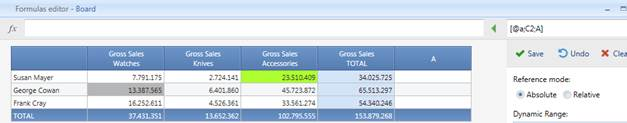The Grey Cell is [@a;C2;A] where “a? is the Block identifier, “C2? George Cowan Code (by row) and “A? the Watches Code (by column).

The Cells in the Formula do not depend on the position (Cell) of the formula

Relative : the Cell is identified by the its shift from the formula position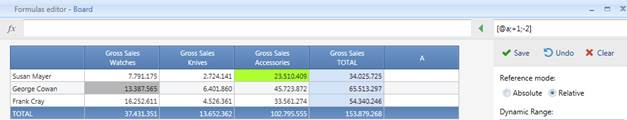The Grey Cell is [@a;+1;-2] where “a" is the Block identifier, +1 is one row below the formula’s row and -2 is two columns before the formula’s column

The Cells in the Formula do depend on the position (Cell) of the formula

NOTES

The Nexel formulas are evaluated after the data-view layout and before the client-side actions. Consequences :

> If you setup a calculated block that uses a "Nexel" block the calculation happens before the Nexel calculation : the result may be not correct

> If you setup a "Nexel" block with a relative references in some formulas, sorting the Blocks does not affect the calculation

## Dynamic Ranges

Ranges provide the capability to include large sets of Cells as Input for Function Calculations. Some Functions as Average make sense only on Range of Cells.

Dynamic Range drop down list facilitates the creation of Ranges. The Dynamic Range syntax has two attributes: action and direction.

Actions can be

·         None : No Action is taken

·         Range: It Selects all the cells in the selected Range

·         Count: It Counts all the cells in the selected Range

·         CountEx: It Counts all the cells in the selected Range non-zero values

·         Point: It selects a single cell depending on the position of the active cell

The “Actions? can be combined with the “Directions?. When using Dynamic Ranges the selected Cells depend not only from the direction attribute but also from the position of the active cell.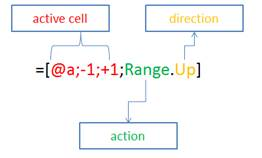·         Whole : The entire range of cells

·         Up: All the Cells above the active cell (included)

·         Down: All the Cells below the active cell (included)

·         Left: All the Cells on the left of the active cell (included)

·         Right: All the Cells on the right of the active cell (included)

·         Vertical : All the Cells of the column of the active cell

·         Horizontal: All the Cells of the row of the active cell

·         Total : The Down Total of the column of the active cell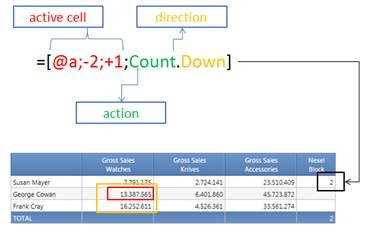Open in Excel

Clicking on this button the Sheet if open in MS Excel. Formulas are not copied as well.

It is possible to add a block in the Layout on the Fly. An empty block (algorithm type) is added to the Layout. The Rule Type must be chosen during the addition action. A New block can also be added from the Formula Editor Panel clicking on the Gear Icon on the block Heading. The new block is created on the right of the existing block.

Allow Write Inplace

Nexel allows to save the results of the Formulas into Info-cubes; this option is similar to execute a data entry on the Layout.

·         Nexel Formulas must be created on a Info-Cube Type block

·         Enable Data Entry must be active on the block

·         Allow Write Inplace button must be active in the Nexel Formula Editor

Data are saved the Data-View Save button in the Sliding Toolbar is clicked.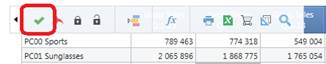Procedure Action Library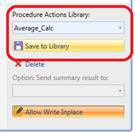It is possible to Save the Current Nexel Sheet as an element of the Action Library.

The Action Library is a property of the Board database.  Such element can be re-use

during the execution of a Capsule and/or Database Procedure.  It can’t be copied

from Database to Database.

NOTES

Nexel Write Back Command : it executes the Nexel Action in Data Entry mode paging throughout all the dimensions of the target cube that are not included in the layout axis. The Procedure Action logics are closer to a recurrent data-entry action than a data-flow and may then be much faster.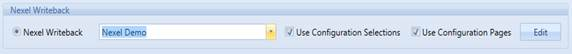Select the Nexel Action from the Library. Choose if the command should use the active Selections and/or Pagers Item  when the Nexel Formulas have been saved or the Procedure Selections.

Edit Button opens the Nexel Action in Formula Editor mode.

How to use Nexel to change the Relationships

Using Nexel it is possible to modify the relationships between two entities depending on the result of a Formula. This allow to maintain relationships and modify them dynamically when some conditions change.

For instance, consider to aggregate Customers in two Categories depending on the value of their Sales:

·         High for the Customers which Sales Value is higher than the Whole Sales Average

·         Low for the Customers which Sales Value is lower than the Whole Sales Average

Setup a layout with the Driver (Gross Sales) and the Customer Category , the latter with Data Entry enabled.

Add in the Formula Editor the calculation of the Average Sales and compare this value with the Customer Sales. The result of this formula is H (High) or L (Low) ; this value is then written back when Save is clicked.

Formula Sample  =if([@a;*;*]>AVERAGE([@a;*;*;Range.Vertical]);"H | High";"L | Low")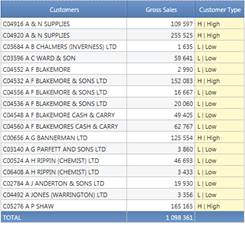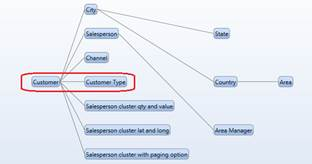How to use of Text Cubes to write Nexel Formulas

Text Cubes can be used to Edit Nexel Formulas. This is useful to create prepackaged Formula Schemas that can be easily reused in different Nexel Sheets.

·         The Layout must have a text Cube Type block (eventually hidden)

·         “Formula In? option must be setup as text Cube Rules where the Text Cube is selected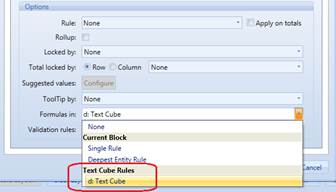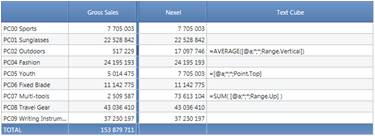NOTES

The Text Infocube must have dimensions coherent with the layout structure (Rows/Columns)

## Nexel and …

### Select/Pagers/Selectors

·         Nexel formulas can refer to single cell or ranges of cells. All those cells MUST be displayed in the Report. It is not allowed to refer to cells that are out of the current Selection.

·         It is possible to use Filters to hide rows: the hidden cells are considered in the execution of the Formula. It is suggested to preview with Hide Zero off for a better troubleshooting.

### Drill Down

·         Drilling down is allowed only for "Single Rule" Formulas: Nexel Layer is applied to the drilled report.

·         If exists at least one "Deepest Entity" Formula, Drill Down option is automatically disabled.

·         Nexel Layer cannot be modified in the drilled report.

Block Format can be setup for Blocks containing Nexel Formulas.

If an Alert is set up on a block where coexist Data and Nexel Formula calculations the latter drives the Alert color coding (not the underlying value)

### Layout Format

Nexel is not supported when Align Vertical is enabled.

### CrossView

Crossview allows to collect data from multiple dataviews inside a screen, it is a very advanced feature that can be used to summarize dataview data in a summary layout.

Usual Nexel syntax is the following:

= F([@Block; Row; Column])

To activate CrossView, just change the syntax in:

= F([#Dataview;@Block; Row; Column])

Where #DataView is the DataView title; when you activate this function, Nexel will just look for the cell reference in the other dataview you specified, so if you are in the third row, Nexel will move into the third row of the other dataview (the one after the “#” sign) and applies ranges and formulas. If the cell does not exist in the other dataview no value will be returned.

Example:

Let’s suppose we have a dataview with sales data by month and another with quantity sold by product and we want to calculate a Summary dataview.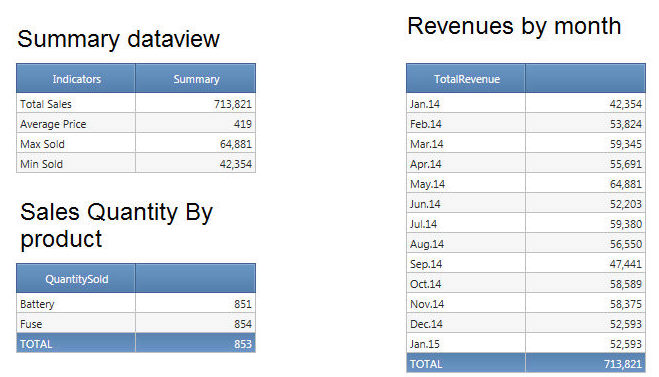Nexel has been used to calculate the summary dataview, let’s see how.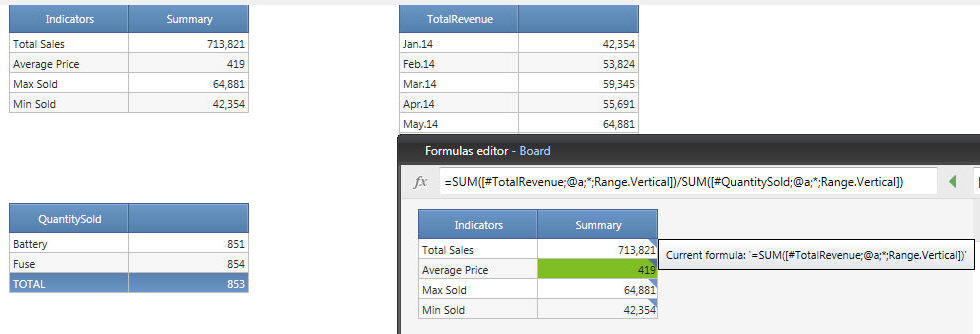We took total value of the two dataviews to calculate average price, while we used TotalRevenue dataview to calculate the other indicators with various formulae (MAX, MIN, SUM).

Please note that the screen must be saved before using CrossView

### Beta Options

"Send Summary Result To" Options are not enabled yet.